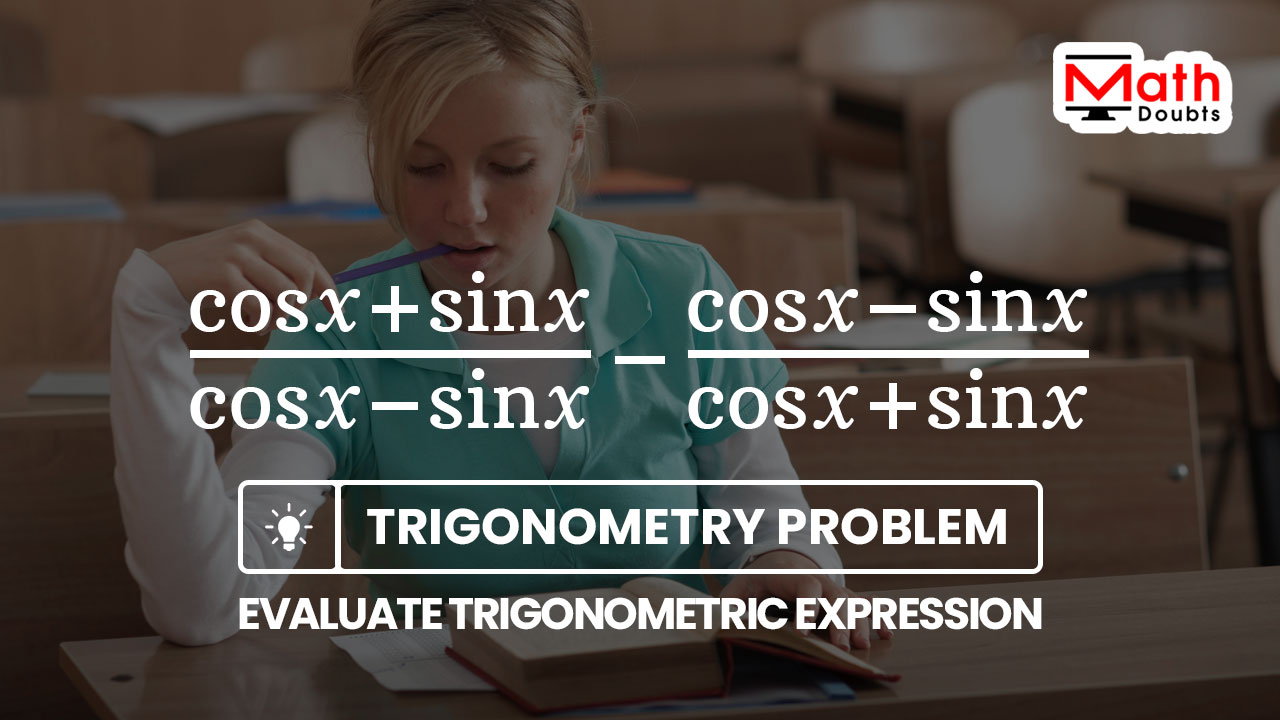# Evaluate $\dfrac{\cos{x}+\sin{x}}{\cos{x}-\sin{x}}$ $-$ $\dfrac{\cos{x}-\sin{x}}{\cos{x}+\sin{x}}$

The trigonometric expressions in this trigonometry problem expresses the difference between two quantities. Each quantity in every term is expressed in rational form by the involvement of two trigonometric functions sine of angle $x$ and cosine of angle $x$.Let’s learn how to evaluate the trigonometric expression the cosine of angle $x$ plus sine of angle $x$ divided by cos of angle $x$ minus sin of angle $x$ minus cos of angle $x$ minus sin of angle $x$ over the cosine of angle $x$ plus sine of angle $x$ by simplifying.

### Converting the Unlike fractions into the Like

Each term in the trigonometric expression is a fraction and compare their denominators. Due to the different denominators, the trigonometric functions in fraction form are unlike fractions.

The unlike fractions in terms of trigonometric functions should be converted into like fractions in terms of trigonometric functions to start the process of the subtraction of the unlike fractions. Now, let’s convert the unlike fractions into like factions firstly in the trigonometric expression.

$=\,\,$ $\bigg(1$ $\times$ $\dfrac{\cos{x}+\sin{x}}{\cos{x}-\sin{x}}\bigg)$ $-$ $\bigg(1$ $\times$ $\dfrac{\cos{x}-\sin{x}}{\cos{x}+\sin{x}}\bigg)$

Now, insert the expression $\cos{x}+\sin{x}$ in the first term and also insert the expression $\cos{x}-\sin{x}$ in the second term to make both terms have the same denominator.

$=\,\,$ $\bigg(\dfrac{\cos{x}+\sin{x}}{\cos{x}+\sin{x}}$ $\times$ $\dfrac{\cos{x}+\sin{x}}{\cos{x}-\sin{x}}\bigg)$ $-$ $\bigg(\dfrac{\cos{x}-\sin{x}}{\cos{x}-\sin{x}}$ $\times$ $\dfrac{\cos{x}-\sin{x}}{\cos{x}+\sin{x}}\bigg)$

In each term, two fractions are involved in multiplication. So, let’s multiply the fractions in the trigonometric expression by the multiplication of the fractions.

$=\,\,$ $\dfrac{(\cos{x}+\sin{x}) \times (\cos{x}+\sin{x})}{(\cos{x}+\sin{x}) \times (\cos{x}-\sin{x})}$ $-$ $\dfrac{(\cos{x}-\sin{x}) \times (\cos{x}-\sin{x})}{(\cos{x}-\sin{x}) \times (\cos{x}+\sin{x})}$

The expressions involving in multiplication in the numerator of each term are same. Hence, the product of such expressions is written in exponential notation. The expressions involving in multiplication in the denominator have same terms with opposite signs between them. Hence, the product of them can be evaluated by the difference of squares formula.

$=\,\,$ $\dfrac{(\cos{x}+\sin{x})^2}{(\cos{x})^2-(\sin{x})^2}$ $-$ $\dfrac{(\cos{x}-\sin{x})^2}{(\cos{x})^2-(\sin{x})^2}$

The squares of sine and cosine functions are written as the sine and cosine squared of angle in trigonometry.

$=\,\,$ $\dfrac{(\cos{x}+\sin{x})^2}{\cos^2{x}-\sin^2{x}}$ $-$ $\dfrac{(\cos{x}-\sin{x})^2}{\cos^2{x}-\sin^2{x}}$

### Find the difference by subtracting the fractions

The denominators of both terms in the trigonometric expression are same, which means the fractions in both terms are like fractions. So, the difference between them in the trigonometric expression can be evaluated by using the subtraction of the like fractions.

$=\,\,$ $\dfrac{(\cos{x}+\sin{x})^2-(\cos{x}-\sin{x})^2}{\cos^2{x}-\sin^2{x}}$

### Find Trigonometric expression by simplification

Let’s focus on simplifying the rational expression. Firstly, let’s simplify the trigonometric expression in the numerator. The trigonometric expression consists of two terms in which one term is the square of sum of two terms and the second term is the difference between two terms.

So, the square of sum of two functions $\cos{x}$ and $\sin{x}$ can be expanded by the square of sum of two quantities formula. Similarly, the square of difference between two functions $\cos{x}$ and $\sin{x}$ can be expanded by the square of difference formula.

$=\,\,$ $\dfrac{(\cos{x})^2+(\sin{x})^2+2\cos{x}\sin{x}-\big((\cos{x})^2+(\sin{x})^2-2\cos{x}\sin{x}\big)}{\cos^2{x}-\sin^2{x}}$

According to the trigonometry, the squares of cosine and sine of angle $x$ are written as cosine square $x$ and sine square $x$ respectively.

$=\,\,$ $\dfrac{\cos^2{x}+\sin^2{x}+2\cos{x}\sin{x}-(\cos^2{x}+\sin^2{x}-2\cos{x}\sin{x})}{\cos^2{x}-\sin^2{x}}$

The negative sign belongs to the three terms in the fourth term. So, let’s distribute the negative sign to all of them to continue the process of simplifying the trigonometric expression in the numerator.

$=\,\,$ $\dfrac{\cos^2{x}+\sin^2{x}+2\cos{x}\sin{x}-\cos^2{x}-\sin^2{x}+2\cos{x}\sin{x}}{\cos^2{x}-\sin^2{x}}$

Use the commutative property of addition to change the places of the terms in expression and it helps us to write the like terms closer.

$=\,\,$ $\dfrac{\cos^2{x}-\cos^2{x}+\sin^2{x}-\sin^2{x}+2\cos{x}\sin{x}+2\cos{x}\sin{x}}{\cos^2{x}-\sin^2{x}}$

The first two terms and the next two terms have the same functions with opposite signs. So, the difference between them is equal to zero. The last two terms can be added to find their sum.

$=\,\,$ $\dfrac{\cancel{\cos^2{x}}-\cancel{\cos^2{x}}+\cancel{\sin^2{x}}-\cancel{\sin^2{x}}+4\cos{x}\sin{x}}{\cos^2{x}-\sin^2{x}}$

$=\,\,$ $\dfrac{4\cos{x}\sin{x}}{\cos^2{x}-\sin^2{x}}$

The sine and cosine functions are involved in the multiplication and their product represents a double angle identity. So, let’s prepare this expression to transform it into that form.

$=\,\,$ $\dfrac{2 \times 2 \times \cos{x}\sin{x}}{\cos^2{x}-\sin^2{x}}$

$=\,\,$ $\dfrac{2 \times 2 \times \cos{x} \times \sin{x}}{\cos^2{x}-\sin^2{x}}$

Now, use the commutative property of multiplication to interchange the positions of the trigonometric functions $\cos{x}$ and $\sin{x}$ in the numerator.

$=\,\,$ $\dfrac{2 \times 2 \times \sin{x} \times \cos{x}}{\cos^2{x}-\sin^2{x}}$

$=\,\,$ $\dfrac{2 \times 2\sin{x}\cos{x}}{\cos^2{x}-\sin^2{x}}$

The two times the product of sine and cosine functions is the expansion of the sine double angle function. So, the second factor in the numerator can be simplified as $\sin{2x}$ function by sine double angle identity.

$=\,\,$ $\dfrac{2 \times \sin{2x}}{\cos^2{x}-\sin^2{x}}$

Now, let’s focus on simplifying the trigonometric expression in the denominator. It is a trigonometric expression in which the trigonometric functions sine and cosine are in square form and the difference between their squares is the expansion of the cosine double angle function. So, use the cos double angle identity to simplify the expression in denominator as $\cos{2x}$.

$=\,\,$ $\dfrac{2 \times \sin{2x}}{\cos{2x}}$

The trigonometric expressions in both numerator and denominator are in simplified form and there is nothing to simplify them individually. So, it is time to simplify the whole function.

The trigonometric functions sine and cosine have same angle and there is a trigonometric identity to find the quotient of sine by cosine functions. Hence, let’s split the rational function as a product of two fractions by using the inverse operation of the multiplication of the fractions.

$=\,\,$ $\dfrac{2 \times \sin{2x}}{1 \times \cos{2x}}$

$=\,\,$ $\dfrac{2}{1}$ $\times$ $\dfrac{\sin{2x}}{\cos{2x}}$

Now, use the quotient of sine by cosine identity to find the quotient of sine of angle $2x$ divided by the cosine of angle $2x$.

$=\,\,$ $2$ $\times$ $\tan{2x}$

$=\,\,$ $2\tan{2x}$

Latest Math Topics
Jun 26, 2023
Jun 23, 2023

###### Math Questions

The math problems with solutions to learn how to solve a problem.

Learn solutions

Practice now

###### Math Videos

The math videos tutorials with visual graphics to learn every concept.

Watch now

###### Subscribe us

Get the latest math updates from the Math Doubts by subscribing us.